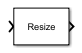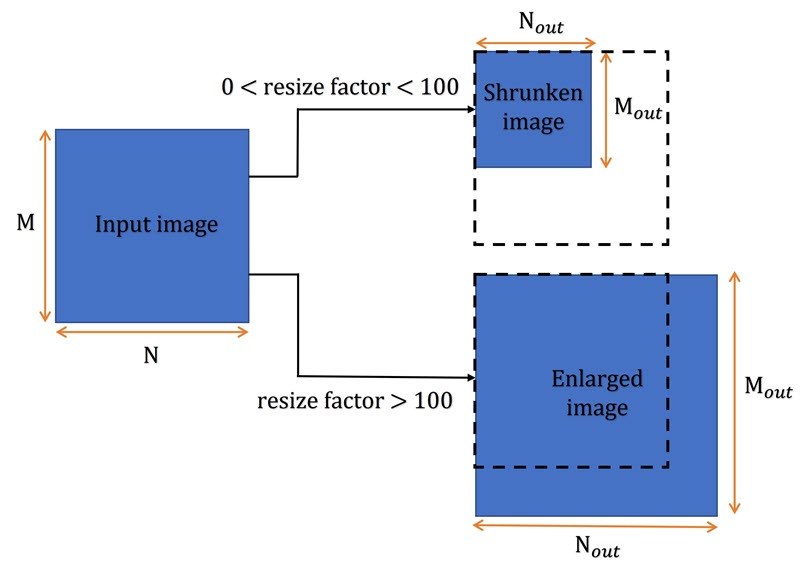# Resize

Enlarge or shrink entire image or region of interest within image

•Libraries:
Computer Vision Toolbox / Geometric Transformations

## Description

The `Resize` block enlarges or shrinks the input image or a region of interest (ROI) within the image. The block resizes along the row dimension followed by the column dimension, or vice-versa.

## Ports

### Input

expand all

Input image or video, specified as an M-by-N matrix, M-by-N-by-T array, M-by-N-by-C array, or M-by-N-by-C-by-T array. T is the number of frames in a video or image sequence, and C is the number of color channels.

Data Types: `single` | `double` | `int8` | `int16` | `int32` | `uint8` | `uint16` | `uint32`

Region of interest, specified as `[x y width height]`, where `[x y]` are the coordinates for the upper-left corner of the region. `width` and `height` specify the width and the height of the region, respectively.

If you specify an input value to the `ROI` port, the `Resize` block applies the transformation only to a specified region in the input image.

#### Dependencies

To enable this port, select the Enable ROI processing parameter.

Data Types: `single` | `double` | `int8` | `int16` | `int32` | `uint8` | `uint16` | `uint32`

### Output

expand all

Resized output image or video, returned as an M-by-N matrix, M-by-N-by-T array, M-by-N-by-C array, or M-by-N-by-C-by-T array. T is the number of frames in a video or image sequence, and C is the number of color channels.

The data type of the resized output image is the same as that of the input image.

Data Types: `single` | `double` | `int8` | `int16` | `int32` | `uint8` | `uint16` | `uint32`

ROI is outside the image region, returned as `0` or `1`.

This table describes the output of the Flag port.

Flag Port OutputDescription
`0`ROI is completely inside the input image.
`1`ROI is completely or partially outside the input image.

#### Dependencies

To enable this port, select these parameters:

• Enable ROI processing

• Output flag indicating if ROI is within image bounds

Data Types: `Boolean`

## Parameters

expand all

Specify aspects of the input image to consider for the transformation as one of these options:

• `Output size as a percentage of input size` — Transforms the input image dimensions such that the output image dimensions are a specified percentage of the input image size. Use the Resize factor in percentage parameter to specify a percentage factor for the entire image or independent percentage factors for the rows and columns of the input image.

• `Number of output columns and preserve aspect ratio` — Transforms the input image column dimension to a specified number of output columns. The block calculates the number of output rows such that the output image has the same aspect ratio as the input image. Use the Number of output columns parameter to specify the number of output columns.

• `Number of output rows and preserve aspect ratio` — Transforms the input image row dimension to a specified number of output rows. The block calculates the number of output columns such that the output image has the same aspect ratio as the input image. Use the Number of output rows parameter to specify the number of output rows.

• `Number of output rows and columns` — Transforms the output image size to a specified number of rows and columns. Use the Number of output rows and columns parameter to specify the number of output rows and columns.

Note

Specifying the number of output rows and columns can change the aspect ratio of the output image.

Specify the percentage resize factor to apply to the input image as one of these options:

• Scalar — Applies the same resize factor to the rows and the columns of the input image.

• Two-element vector value — The first element of the vector specifies the percentage resize factor to apply to the rows of the image, and the second element specifies the percentage resize factor to apply to the columns.

For a scalar Resize factor in percentage value, resize factor, the block calculates the dimensions ```[Mout Nout]``` of the output image using these equations:To resize the input image, specify a resize factor value greater than zero. This table describes the effect of various resize factor values on the input image.

Resize Factor PercentageEffect on Image
`0` < resize factor < `100`The Resize block shrinks the image.
resize factor = `100`Image size is unchanged.
resize factor > `100`The Resize block enlarges the image.#### Dependencies

To enable this parameter, set the Specify parameter value to `Output size as a percentage of input size`.

Specify the number of columns in the output image.

#### Dependencies

To enable this parameter, set the Specify parameter value to ```Number of output columns and preserve aspect ratio```.

Specify the number of rows in the output image.

#### Dependencies

To enable this parameter, set the Specify parameter value to `Number of output rows and preserve aspect ratio`.

Specify the number of rows and columns in the output image, specified as a two-element vector. The first element specifies the number of rows in the output image, and the second element is the number of columns.

#### Dependencies

To enable this parameter, set the Specify parameter value to `Number of output rows and columns`.

Specify the method for interpolating the pixel values of the resized image as one of these options:

• `Nearest neighbor` — The Resize block uses the value of 1 nearest pixel for the new pixel value.

• `Bilinear` — The new pixel value is the weighted average of the 4 nearest pixel values.

• `Bicubic` — The new pixel value is the weighted average of the 16 nearest pixel values.

• `Lanczos2` — The new pixel value is the weighted average of the 16 nearest pixel values, calculated using the Lanczos-2 kernel.

• `Lanczos3` — The new pixel value is the weighted average of the 36 nearest pixel values, calculated using the Lanczos-3 kernel.

The number of pixels the block considers for interpolation affects the complexity and accuracy of the computation. The `Nearest neighbor` interpolation, which uses the fewest pixels, is the most computationally efficient and least accurate while the interpolation with `Lanczos3` kernel is the least computationally efficient and the most accurate. For more information about the interpolation methods and kernels, see the More About section and the Create and Compare Resizing Interpolation Kernels example.

Select this parameter to perform low-pass filtering on the input image before shrinking it. Selecting this parameter can prevent aliasing in the output image when the value of the Resize factor in percentage parameter is between `0` and `100`.

Select this parameter to enable the ROI input port, for specifying a region of interest to resize in the input image.

#### Dependencies

To enable this parameter:

• Set the Specify parameter to ```Number of output rows and columns```.

• Set the Interpolation method to one of these options:

• `Nearest neighbor`

• `Bilinear`

• `Bicubic`

• Clear the Perform antialiasing when resize factor is between 0 and 100 parameter.

Select this parameter to enable the Flag port which indicates if the specified ROI value is completely or partially outside the range of the input image dimensions.

#### Dependencies

To enable this parameter, select the Enable ROI processing parameter.

## Block Characteristics

 Data Types `Boolean` | `double` | `fixed point` | `integer` | `single` Multidimensional Signals `yes` Variable-Size Signals `no`